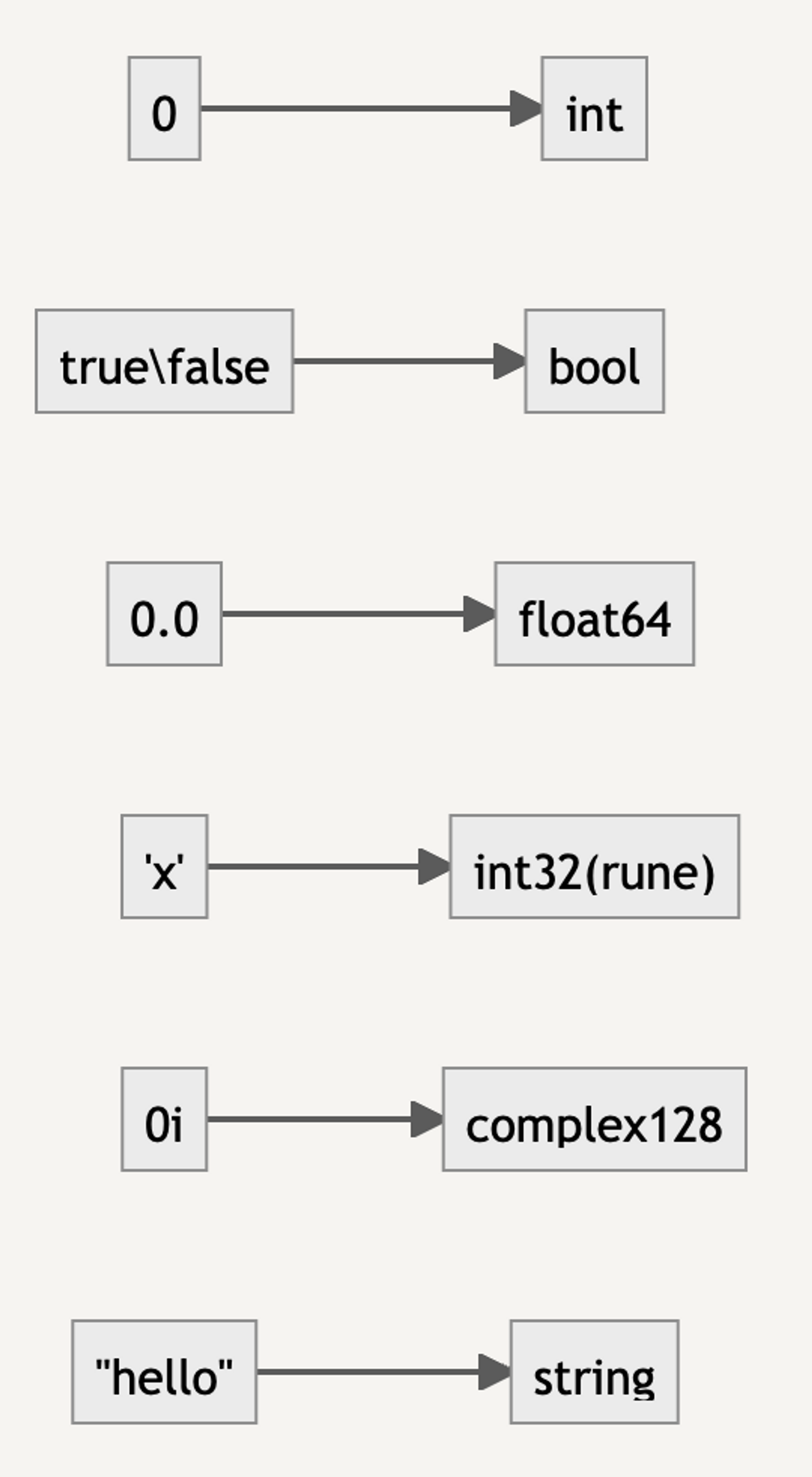`````` 1 2 3 4 5 6 7 8 9 10 11 12 13 `````` ``````import ( "time" "math/rand" ) // invalid operation: rand.Intn(10) * 1000 * time.Millisecond (mismatched types int and time.Duration) time.Sleep(rand.Intn(10) * 1000 * time.Millisecond) ❌ // 🤔 make sense. time.Sleep(time.Duration(rand.Intn(10) * 1000) * time.Millisecond) ✅ // wtf ?! time.Sleep(1000 * time.Millisecond) ✅ ``````

Look at this simple example above.

• The first error is easy to understand: integers cannot be multiplied with `time.Duration`
• The second example fixes the problem, as expected
• The third example raises the question, why is `1000 * time.Millisecond` not a problem? Is `1000` also an integer?

Don’t panic, take a closer look at the difference between `rand.Intn(10) * 1000` and `1000`: the former is a variable, the type has already been determined, it is reasonable to fail to compile; while the latter is a constant, the type is not `int`, belongs to `untyped constants`, the compiler will try to convert it to `time.Duration`.

This aroused my curiosity, what if I write a `float` constant?

 ``````1 2 `````` ``````// (untyped float constant) truncated to int64 time.Sleep(1000.1 * time.Millisecond) ❌ ``````

Sure enough, it doesn’t work. So what exactly are the rules for type conversion of untyped constants?

First of all, each type of constant corresponds to a default type.Then, take a look at the definition of `time.Duration`.

 `````` 1 2 3 4 5 6 7 8 9 10 `````` ``````type Duration int64 const ( Nanosecond Duration = 1 Microsecond = 1000 * Nanosecond Millisecond = 1000 * Microsecond Second = 1000 * Millisecond Minute = 60 * Second Hour = 60 * Minute ) ``````

That means the default type of `1000` is `int` and the compiler will try to do a type conversion `int``time.Duration` and `int64` and `int` are fully compatible, so the compilation passes.

WTF，Go…